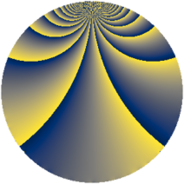# Properties

 Label 3528.1.cgLevel $3528$ Weight $1$ Character orbit 3528.cg Rep. character $\chi_{3528}(2059,\cdot)$ Character field $\Q(\zeta_{6})$ Dimension $22$ Newform subspaces $5$ Sturm bound $672$ Trace bound $3$

# Related objects

## Defining parameters

 Level: $$N$$ $$=$$ $$3528 = 2^{3} \cdot 3^{2} \cdot 7^{2}$$ Weight: $$k$$ $$=$$ $$1$$ Character orbit: $$[\chi]$$ $$=$$ 3528.cg (of order $$6$$ and degree $$2$$) Character conductor: $$\operatorname{cond}(\chi)$$ $$=$$ $$72$$ Character field: $$\Q(\zeta_{6})$$ Newform subspaces: $$5$$ Sturm bound: $$672$$ Trace bound: $$3$$

## Dimensions

The following table gives the dimensions of various subspaces of $$M_{1}(3528, [\chi])$$.

Total New Old
Modular forms 60 42 18
Cusp forms 28 22 6
Eisenstein series 32 20 12

The following table gives the dimensions of subspaces with specified projective image type.

$$D_n$$ $$A_4$$ $$S_4$$ $$A_5$$
Dimension 14 8 0 0

## Trace form

 $$22q + q^{2} + q^{3} - 3q^{4} + q^{6} - 2q^{8} - 3q^{9} + O(q^{10})$$ $$22q + q^{2} + q^{3} - 3q^{4} + q^{6} - 2q^{8} - 3q^{9} + 3q^{11} - 2q^{12} - 11q^{16} + 2q^{17} - 2q^{18} + 2q^{19} - q^{22} + q^{24} - 7q^{25} - 2q^{27} - 4q^{30} + q^{32} - q^{33} - q^{34} - 7q^{36} - q^{38} - q^{41} + 3q^{43} + 10q^{44} - 8q^{46} + q^{48} + q^{50} - 5q^{51} + q^{54} + 3q^{57} + 4q^{58} - q^{59} + 6q^{64} + 4q^{65} + 2q^{66} - q^{67} - q^{68} + q^{72} + 2q^{73} + 4q^{74} + q^{75} - q^{76} - 4q^{78} - 3q^{81} + 2q^{82} + 2q^{83} - q^{86} - q^{88} - 4q^{89} - 2q^{96} - q^{97} - 2q^{99} + O(q^{100})$$

## Decomposition of $$S_{1}^{\mathrm{new}}(3528, [\chi])$$ into newform subspaces

Label Dim. $$A$$ Field Image CM RM Traces $q$-expansion
$$a_2$$ $$a_3$$ $$a_5$$ $$a_7$$
3528.1.cg.a $$2$$ $$1.761$$ $$\Q(\sqrt{-3})$$ $$D_{3}$$ $$\Q(\sqrt{-2})$$ None $$-1$$ $$1$$ $$0$$ $$0$$ $$q-\zeta_{6}q^{2}+\zeta_{6}q^{3}+\zeta_{6}^{2}q^{4}-\zeta_{6}^{2}q^{6}+\cdots$$
3528.1.cg.b $$4$$ $$1.761$$ $$\Q(\zeta_{12})$$ $$D_{6}$$ $$\Q(\sqrt{-2})$$ None $$-2$$ $$0$$ $$0$$ $$0$$ $$q+\zeta_{12}^{4}q^{2}-\zeta_{12}q^{3}-\zeta_{12}^{2}q^{4}-\zeta_{12}^{5}q^{6}+\cdots$$
3528.1.cg.c $$4$$ $$1.761$$ $$\Q(\zeta_{12})$$ $$A_{4}$$ None None $$0$$ $$-2$$ $$0$$ $$0$$ $$q-\zeta_{12}q^{2}-\zeta_{12}^{2}q^{3}+\zeta_{12}^{2}q^{4}+\zeta_{12}^{5}q^{5}+\cdots$$
3528.1.cg.d $$4$$ $$1.761$$ $$\Q(\zeta_{12})$$ $$A_{4}$$ None None $$0$$ $$2$$ $$0$$ $$0$$ $$q-\zeta_{12}q^{2}+\zeta_{12}^{2}q^{3}+\zeta_{12}^{2}q^{4}-\zeta_{12}^{5}q^{5}+\cdots$$
3528.1.cg.e $$8$$ $$1.761$$ $$\Q(\zeta_{24})$$ $$D_{12}$$ $$\Q(\sqrt{-2})$$ None $$4$$ $$0$$ $$0$$ $$0$$ $$q-\zeta_{24}^{8}q^{2}-\zeta_{24}^{5}q^{3}-\zeta_{24}^{4}q^{4}+\cdots$$

## Decomposition of $$S_{1}^{\mathrm{old}}(3528, [\chi])$$ into lower level spaces

$$S_{1}^{\mathrm{old}}(3528, [\chi]) \cong$$ $$S_{1}^{\mathrm{new}}(72, [\chi])$$$$^{\oplus 3}$$